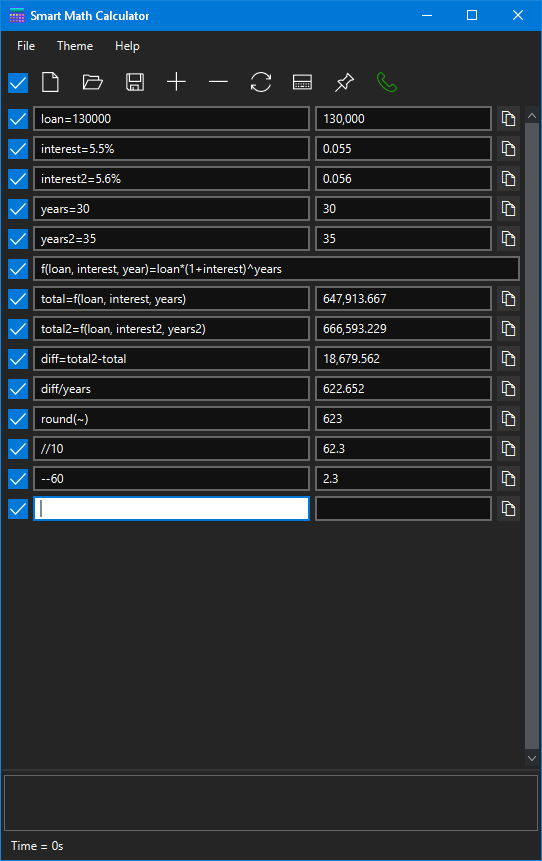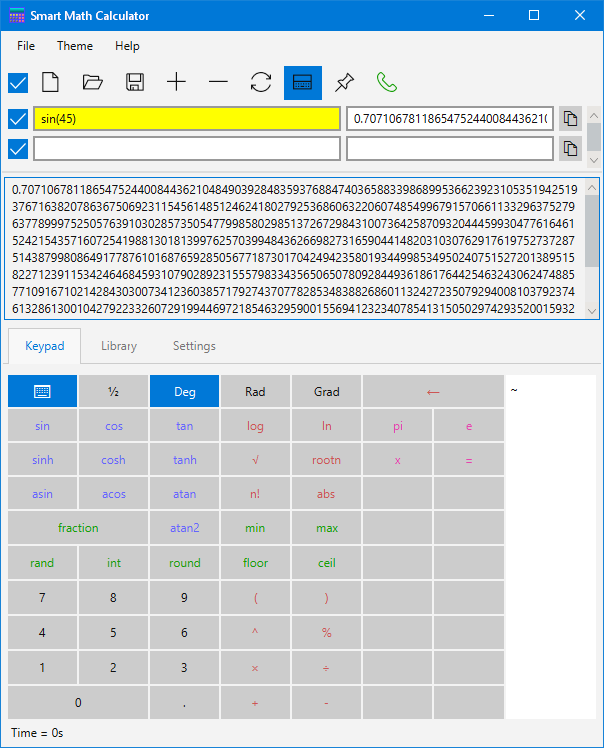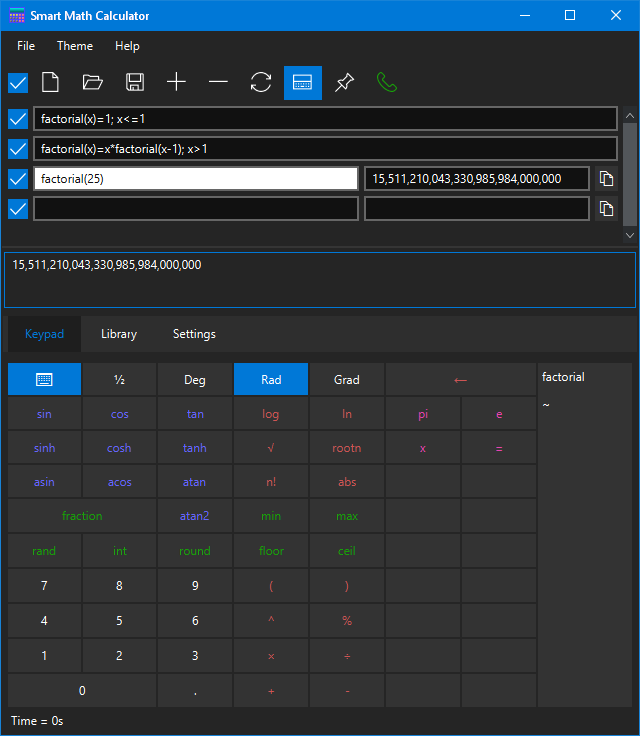Smart Math Calculator

Calculate multiple math expressions simultaneously and save the result.
Save results in variables with up to a million digits precision.
Can be installed in any Windows, Mac or Linux computer.

Multiple Calculations

Calculate multiple math expressions and keep them in separate lines, so you can edit them later. The results are instantly recalculated as you edit the math expressions.

Variables and Functions

Save the result of calculations in variables. Define your own functions with as many parameters as you like. Add condition to functions or turn them into recursive functions.

Unlimited Precisions

Compute the result of math expressions with up to one million digits precision. That should be enough precision for even the most die-hard mathematicians and physicists.

The computation capabilities of Smart Math Calculator includes:

• Fast auto-compute while typing
• Multiple simultaneous expressions
• High-precision (Up to 1 million digits)
• Option to keep in system-tray for fast pop-up
• Solve equations
• Define variables
• Define functions
• Define recursive functions
• Fraction calculator
• Arbitrary bases (binary, oct, hex, etc.)
• Auto-save current session
• Save calculation sessions in files
• Open previously saved calculation sessions
• Print calculations
• Over 20 built-in functions and library

This software can be installed on Windows, Mac or Linux computers. Download the free version to start calculating now.